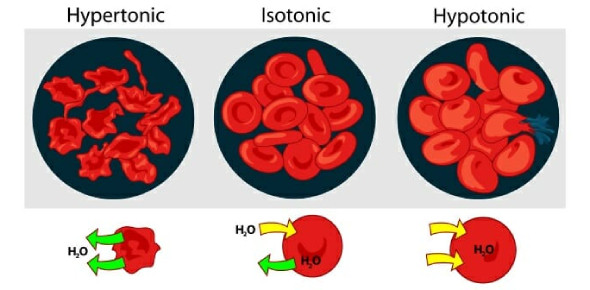# Quiz: Biology Tonicity Practice Test

19 Questions | Total Attempts: 2493SettingsQuiz: biology tonicity practice test. Osmosis, in simple terms, is the movement of solvents from a higher solute concentration to the less one through a semipermeable membrane. In the quiz below, you will get to test your understanding of different tonic solutions and how a solute is transferred. How about you try it out and see what you may learn about osmosis as a whole.

• 1.
In a hypotonic solution the total molar concentration of all dissolved solute particles is greater than that of another solution
• A.

True

• B.

False

• 2.
When a cell is exposed to hypotonic conditions, there is net water movement into the cell?
• A.

True

• B.

False

• 3.
Hypotonic solution means more solute and less water.
• A.

True

• B.

False

• 4.
Plant cells take water by  Osmosis in a hypertonic solution
• A.

True

• B.

False

• 5.
___________ prevents a plant cell from bursting when kept in a hypotonic solution.
• 6.
What is the Movement of WATER IN and OUT of the cell membrane?
• A.

Osmosis

• B.

Diffusion

• C.

Hypotonic

• D.

Isotonic

• 7.
Click all the boxes that apply. The movement of molecules from an area of HIGHer Concentration TO an area of LOWer Concentration
• A.

Diffusion

• B.

Osmosis

• C.

Hypotonic

• D.

Isotonic

• 8.
EQUAL AMOUNT OF SOLUTE AND SOLVENT IN AND OUT OF THE CELL Equal amount of solute and solvent in and out of a cell
• A.

Isotonic

• B.

Hypotonic

• C.

Hypertonic

• D.

None of the above

• 9.
Why do your fingers Prune when in the water for a long time?
• A.

Osmosis

• B.

Diffusion

• C.

Hypotonic

• D.

Hypertonic

• 10.
Water is a hypotonic solution
• A.

True

• B.

False

• 11.
Soda drinks are what kind of solution?
• A.

Hypertonic

• B.

Hypotonic

• C.

Isotonic

• D.

None

• 12.
Blood is what kind of solution?
• A.

Isotonic

• B.

Hypertonic

• C.

Hypotonic

• D.

None

• 13.
What kind of solution is it if the cell grows when placed in the solution?
• A.

Hypotonic

• B.

Hypertonic

• C.

Isotonic

• D.

I didn't watch my lesson

• 14.
A ________ solution would cause a cell to shrink.
• 15.
A hypertonic solution would cause
• A.

Water to enter the cell

• B.

Water to leave the cell

• C.

Water to evaporate

• 16.
A HYPOTONIC solution has a ______________ concentration of solute (dissolved stuff) than inside the cell.
• A.

Lower

• B.

Higher

• C.

Equal

• 17.
If a cell is placed in a HYPOTONIC solution, the water will move into the cell, causing it to swell.
• A.

True

• B.

False

• 18.
Movement of anything from an area of high concentration to low concentration.
• A.

Diffusion

• B.

Osmosis

• C.

Hypertonic

Related TopicsBack to top